# KSEEB SSLC Class 10 Maths Solutions Chapter 10 Quadratic Equations Ex 10.4

KSEEB SSLC Class 10 Maths Solutions Chapter 10 Quadratic Equations Ex 10.4 are part of KSEEB SSLC Class 10 Maths Solutions. Here we have given Karnataka SSLC Class 10 Maths Solutions Chapter 10 Quadratic Equations Exercise 10.4.

## Karnataka SSLC Class 10 Maths Solutions Chapter 10 Quadratic Equations Exercise 10.4

Question 1.
Find the nature of the roots of the following quadratic equations. If the real roots exist, find them :
(i) 2x2 – 3x + 5 = 0
(ii) 3x2 – $$4 \sqrt{3} x$$ + 4 = 0
(iii) 2x2 – 6x + 3 = 0
Solution:
i) 2x2 – 3x + 5 = 0
It is in the form of ax2 + bx + c = 0
a = 2, b = – 3 and c = 5
Nature of roots = b2 – 4ac
Discriminant = (- 3)2 – 4 × (2)(5)
= 9 – 40
= – 31
Discriminant = – 31 < 0
The given Quadratic equation has no real roots.

(ii) 3x2 – $$4 \sqrt{3} x$$ + 4 = 0
Here, a = 3, b = $$4 \sqrt{3}$$, c = 4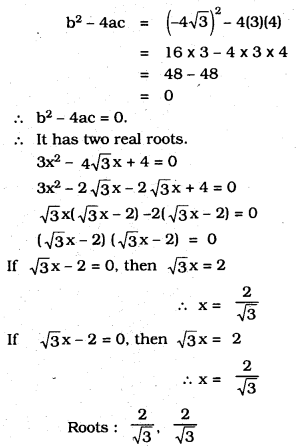(iii) 2x2 – 6x + 3 = 0
Here, a = 2, b = -6, c = 3
b2 – 4ac = (-6)2 – 4(2)(3)
= 36 – 24 = 12
∴ b2 – 4ac = 12 > 0.
∴ It has two distinct roots.
2x2 – 6x + 3 = 0
Here, a = 2, b = -3, c = 3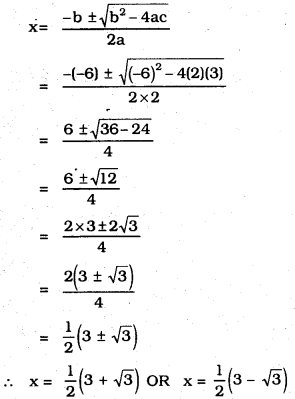Question 2.
Find the values of k for each of the following quadratic equations, so that they have two equal roots
(i) 2x2 + kx+3 = 0
(ii) kx(x – 2) + 6 = 0
Solution:
(i) Comparing the given quadratic equation with ax2+ bx + c = 0, we get a = 2,b = k,c = 3
∴ b2 – 4ac = (k)2 – 4(2)(3) = k2 – 24
∵ For a quadratic equation to have equal roots, b2 – 4ac = 0(ii) kx(x – 2) + 6 = 0 ⇒ kx2 – 2kx + 6 = 0
Comparing kx2 – 2kx + 6 = 0 with ax2 + bx + c = 0, we get
a = k, b = -2k, c = 6
∴  b2 – 4ac = (-2k)2 – 4(k)(6) = 4k2 – 24k
Since, the roots are equal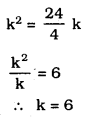But k cannot be 0, otherwise, the given equation is not quadratic. Thus, the required value of k is 6.Question 3.
Is it possible to design a rectangular mango grove whose length is twice its breadth, and the area is 800 m2 ? If so, find its length and breadth.Solution:
Let the breadth of rectangular mango grove is ‘x’ and length of rectangular mango grove is 2x
Area of rectangular mango grove = 800 m2
x (2x)= 800
2x2 = 800
x2 = $$\frac{800}{2}$$ = 400
x = ± $$\sqrt{400}$$
x = ± 20m
∴ breadth of mango grove x = 20 m
length of mango grove = 2x = 2 × 20 = 40 m
It is possible to construct a rectangular mango grove of length 40m and breadth 20m.Question 4.
Is the following situation possible? If so, determine their present ages. The sum of the ages of two friends is 20 years. Four years ago, the product of their ages in years was 48.
Solution:
Let the age of one friend = x years
∴ Age of other friend = (20 – x) years
Four years ago,
Age of one friend = (x – 4) years
Age of other friend = (20 – x – 4) years
= (16 -x) years
According to the condition,
(x – 4) × (16 – x) = 48
⇒ 16x – 64 – x2 + 4x = 48
⇒ – x2 + 20x – 64 – 48 = 0
⇒ -x2 + 20x – 112 = 0
⇒ x2 – 20x + 112 = 0 …(1)
Comparing equation (1) with ax2 + bx + c = 0, we get a = 1, b = – 20 and c = 112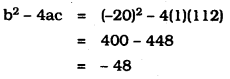Since, b2 – 4ac is less than 0.
∴ The quadratic equation (1) has no real roots.
Thus, the given situation is not possible.Question 5.
Is it possible to design a rectangular park of perimeter 80 m. and area 400 m2? If so, find its length and breadth.
Solution: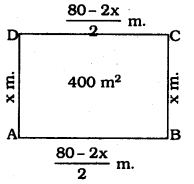Let the length of rectangular park is ‘x’
Perimeter of the rectangular park = 80m
2 (length + breadth) = 80
length + breadth = $$\frac{80}{2}$$ = 40
area of rectangle = l × b
x (40 – x) = 400
40x – x2 = 400
x2 – 40x + 400 = 0
x2 – 20x – 20x + 400 = 0
x (x – 20) – 20 (x – 20) = 0
(x – 20) (x – 20) = 0
x – 20 (or) x – 20 = 0
x = 20 m
length of rectangular park = 20 m and breadth of rectangular park = (40 – x)
= 40 – 20 = 20m
∴ It is possible to design rectangular park of length 20 m and breadth is 20 m.
∴ The park is a square having 20m side.

We hope the given KSEEB SSLC Class 10 Maths Solutions Chapter 10 Quadratic Equations Ex 10.4 will help you. If you have any query regarding Karnataka SSLC Class 10 Maths Solutions Chapter 10 Quadratic Equations Exercise 10.4, drop a comment below and we will get back to you at the earliest

error: Content is protected !!